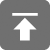# 工学1号馆

home

## 冒泡排序算法

Wu Yudong    July 25, 2016     Algorithm   3481. 比较相邻的元素。如果第一个比第二个大，就交换他们两个。
2. 对每一对相邻元素作同样的工作，从开始第一对到结尾的最后一对。这样最后的元素应该会是最大的数。
3. 针对所有的元素重复以上的步骤，除了最后一个。
4. 持续每次对越来越少的元素重复上面的步骤，直到没有任何一对数字需要比较。

#include<stdio.h>
#include<stdlib.h>

void swap(void *vp1, void *vp2, int size){
char buffer[size];
memcpy(buffer, vp1, size);
memcpy(vp1, vp2, size);
memcpy(vp2, buffer, size);
}

int cmp_int(const void *a , const void *b )
{
return *(int *)a - *(int *)b;
}

int cmp_double(const void *a , const void *b )
{
return *(double *)a > *(double *)b ? 1 : -1;
}

void bubbleSort(void *base, int n, int elemsize, int(*cmp)(const void *, const void *))
{
char *q = (char *)base;
char *p = (char *)base + n * elemsize;
while(p > q) {
for(; q != p - elemsize; q += elemsize) {
if(cmp(q, q + elemsize) > 0) {
swap(q, q + elemsize, elemsize);
}
}
q = (char *)base;
p -= elemsize;
}
}

int main(void)
{
//测试数据
int arr1[] = {5, 4, 1, 3, 6, 12, 8, 22, 34,76};
//冒泡排序
bubbleSort(arr1, 10, sizeof(int), cmp_int);
//打印排序结果
int i;
for(i = 0; i < 10; i++)
printf("%d ", arr1[i]);
printf("\n");
double arr2[] = {5.4, 4.8, 1.2, 3.4, 6.7, 12.12, 8.6, 22.12, 34.5, 76.3};
bubbleSort(arr2, 10, sizeof(double), cmp_double);
for(i = 0; i < 10; i++)
printf("%.2f ", arr2[i]);
printf("\n");
}#include <stdio.h>

void bubbleSort(int arr[], int count)
{
int i = count, j;
int temp;
while(i > 0) {
for(j = 0; j < i - 1; j++) {
if(arr[j] > arr[j + 1]){
temp = arr[j];
arr[j] = arr[j + 1];
arr[j + 1] = temp;
}
}
i--;
}

}
int main()
{
//测试数据
int arr[] = {5, 4, 1, 3, 6};
//冒泡排序
bubbleSort(arr, 5);
//打印排序结果
for(int i = 0; i < 5; i++)
printf("%4d", arr[i]);
}

void bubbleSort(int d[], int size)  //假定两两交换发生在数组最后的两个位置
{
int exchange = size - 1;
while(exchange) {
//记录下发生数据交换的位置
int bound = exchange;
exchange = 0;   //假定本趟比较没有数据交换
for(int i = 0; i < bound; i++) {
if(d[i] > d[i + 1]) {
swap(&d[i], &d[i+1]);
exchange = i;
}
}
}
}